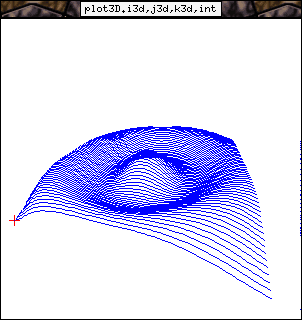# The 3D plotline operator

Agat can be used like a plotter of 3D functions.
Here is an example of a function representation. Here, the agat script is used to compute values to plot. In a more realistic case these values would be computed by your algorithm and send directly into the 3d graphic operator.

## The C code

The same as for the plotline example.

## The Agat code

```let PI= atan(1)*4;

-- i is an extern stream giving each integer between 1 and nb
-- nb is a stream

------------
-- map 3d --
------------

let i3d= map{pan}(i,+-> ceil(i/pan) /pan *4*PI -2*PI
|*,nb^-> pan := sqrt(nb),
null
) i,nb;

let j3d= map{pan}(i,+-> ceil(fmod(i,pan)) /pan *4*PI -2*PI
|*,nb^-> pan := sqrt(nb),
null
) i,nb;

let int= map{pan}(i,+-> if (floor(fmod(i,pan)) == 0) then 1 else 0 endif
|*,nb^-> pan := sqrt(nb),
null
) i,nb;

-- bessel
let k3d= map(i,j-> cos(sqrt(i*i+j*j)))i3d,j3d;

plot3dlineint(i3d,j3d,k3d,int);
```

## The result of the executionAnd you can interact with this view using mouse!